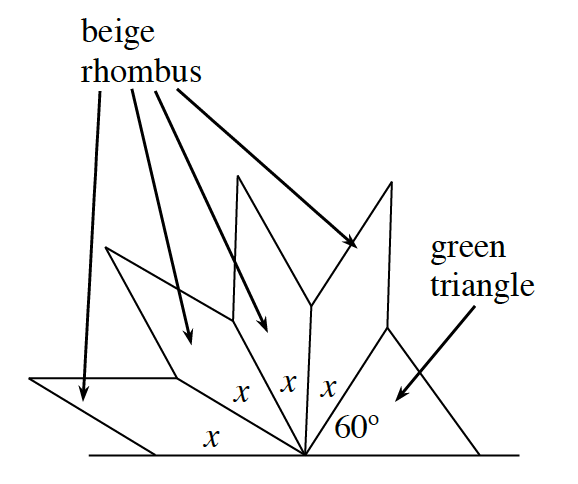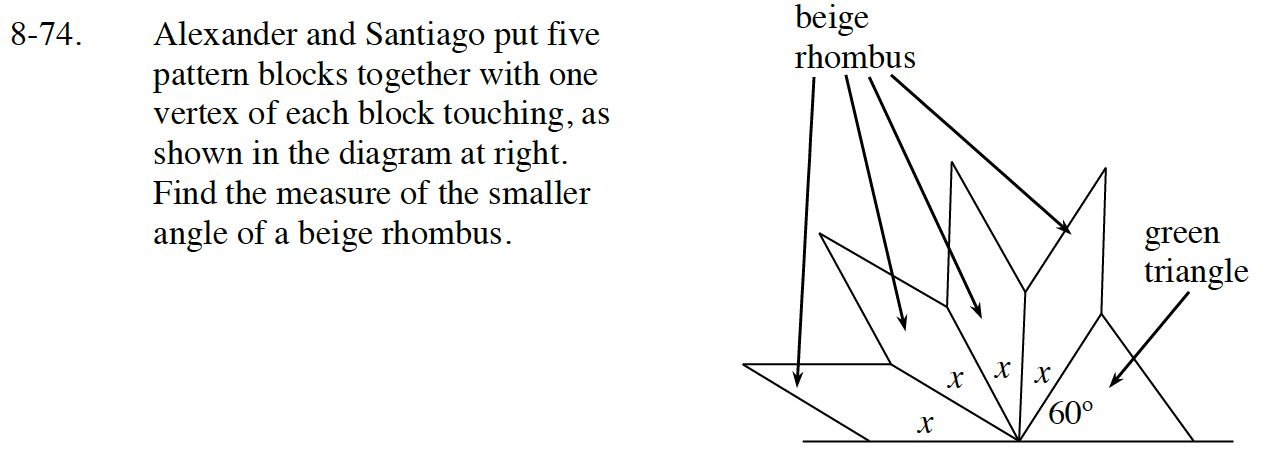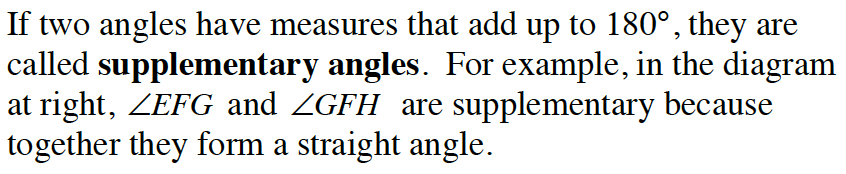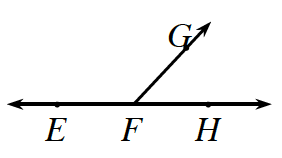Home > CC2 > Chapter 8 > Lesson 8.3.2 > Problem8-74

8-74.Alexander and Santiago put five pattern blocks together with one vertex of each block touching, as shown in the diagram at right. Find the measure of the smaller angle of a beige rhombus. Homework Help ✎Look at the information below from the Math Box in Lesson 8.3.2.
How are the angles in the diagram similar to the angles in the example?Because all the angles add up to 180°, you can set up the equation:
x + x + x + x + 60° = 180°

Combine like terms:
4x + 60° = 180°

Solve for x.

x = 30°